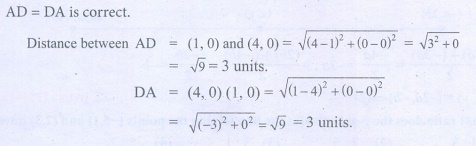Home | | Maths 9th std | Exercise 5.1: Devising a Coordinate System

# Exercise 5.1: Devising a Coordinate System

Maths : Coordinate Geometry: Book Back, Exercise, Example Numerical Question with Answers, Solution: Exercise 5.1: Mapping the Plane, Devising a Coordinate System

Exercise 5.1

1. Plot the following points in the coordinate system and identify the quadrants P(–7,6), Q(7,–2), R(–6,–7), S(3,5) and T(3,9)2. Write down the abscissa and ordinate of the following from fig 5.11.

(i) P

(ii) Q

(iii) R

(iv) S3. Plot the following points in the coordinate plane and join them. What is your conclusion about the resulting figure?

(i) (–5,3) (–1,3) (0,3) (5,3)

(ii) (0,–4) (0,–2) (0,4) (0,5)4. Plot the following points in the coordinate plane. Join them in order. What type of geometrical shape is formed?

(i) (0,0) (–4,0) (–4,–4) (0,–4)

(ii) (–3,3) (2,3) (–6,–1) (5,–1)Activity – 1

Plot the following points on a graph sheet by taking the scale as 1cm = 1 unit.

Find how far the points are from each other?

A (1, 0) and D (4, 0). Find AD and also DA.

You plot another set of points and verify your Result.Solution:Tags : Numerical Problems with Answers, Solution | Coordinate Geometry | Maths , 9th Maths : UNIT 5 : Coordinate Geometry
Study Material, Lecturing Notes, Assignment, Reference, Wiki description explanation, brief detail
9th Maths : UNIT 5 : Coordinate Geometry : Exercise 5.1: Devising a Coordinate System | Numerical Problems with Answers, Solution | Coordinate Geometry | Maths